# How would I determine the mmoles of phosphoric acid used in an experiment? experiment details: phosphoric...

How would I determine the mmoles of phosphoric acid used in an experiment?

experiment details:

phosphoric acid 85% in water
amount used: 1mL
molecular weight: ?
density: ?

Given that:-

Phosphoric acid: 85% in water (w/w)

(w/w means 85 g of phosphoric acid in 100 g of water)

Amount of solution used: 1ml

Molecular weight of phosphoric acid: 97.994 g/mol

Density of phosphoric acid: 1.88 g/ml

​​​​​Mass of phosphoric acid used to make solution is: 85g

Therefore volume of phosohoric acid used is: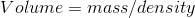= 85/1.88

= 45.21 ml

Also as the density of water is 1 g/ml therefore volume of water used is 100 only

Hence, total volume of solution is: 100 ml water+ 45.21 ml phosohoric acid = 145.21 ml

Now,

No. of moles of phosohoric acid in 145.21 ml of solution is given by: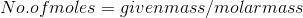= 85/97.994

= 0.867 moles

No. of moles of phosphoric acid in 1 ml of solution is:

= 0.867/145.21

= 0.00597 moles

Therefore no. of mmoles of phosohoric acid in 1 ml solution is:

= 0.00597/1000

= 0.00000597

= 5.97 * 10-6 mmoles

​​​​​​

#### Earn Coin

Coins can be redeemed for fabulous gifts.

Similar Homework Help Questions
• ### Barium hydroxide reacts with phosphoric acid to form barium phosphate and water. How many mL of...

Barium hydroxide reacts with phosphoric acid to form barium phosphate and water. How many mL of 0.2195 M barium hydroxide would be required to titrate 12.57 mL of phosphoric acid? (Density of ohosphoric acid is 1.834 g/mL.) >> answer should be 1608 mL

• ### What famous equation was used to determine the first and second K_a for phosphoric acid?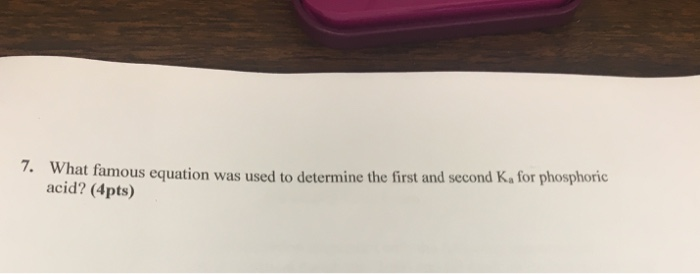What famous equation was used to determine the first and second K_a for phosphoric acid?

• ### If concentrated phosphoric acid is 14.7 M, how many ml of acid would you use to...

If concentrated phosphoric acid is 14.7 M, how many ml of acid would you use to make 400 ml of 2N phosphoric acid?

• ### I am writing a prelab for this experiment, but am struggling on how to figure out...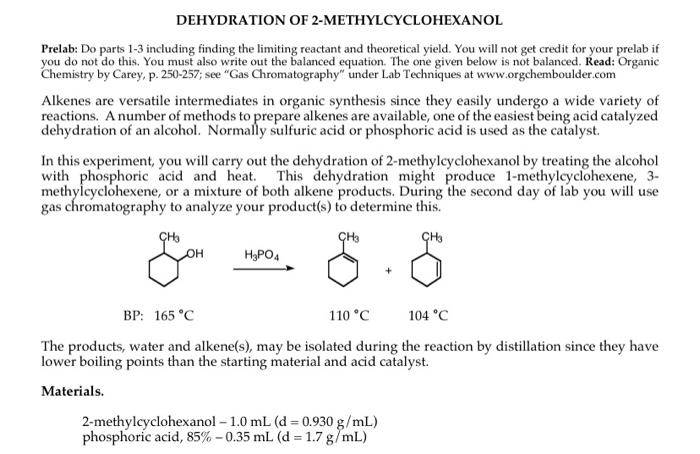I am writing a prelab for this experiment, but am struggling on how to figure out what the balanced chemical equation is. Any help is much appreciated, thank you! DEHYDRATION OF 2-METHYLCYCLOHEXANOL Prelab: Do parts 1-3 including finding the limiting reactant and theoretical yield. You will not get credit for your prelab if you do not do this. You must also write out the balanced equation. The one given below is not balanced. Read: Organic Chemistry by Carey, p. 250-257;...

• ### answer it all please BS132 Experiment 3 2018-2019 The limiting reagent is the reagent which will...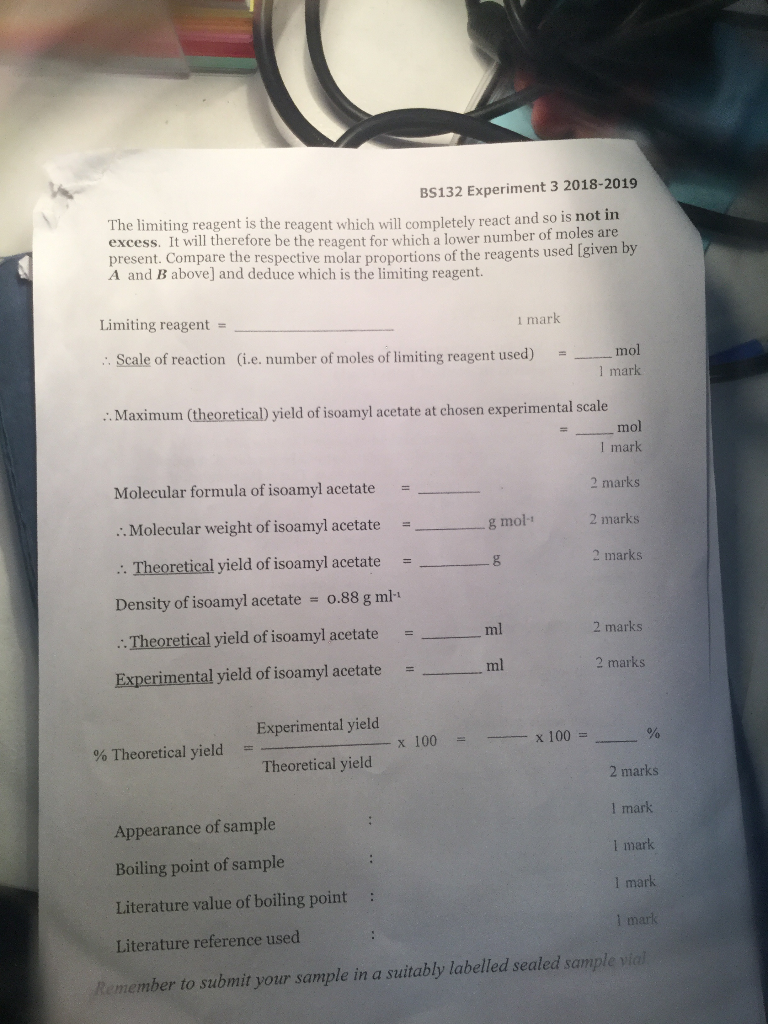answer it all please BS132 Experiment 3 2018-2019 The limiting reagent is the reagent which will completely react and so iIs excess. It will therefore be the reagent for which a lower number o present. Compare the respective molar proportions of the reagents used A and B above] and deduce which is the limiting reagent not in f moles are Igiven by Limiting reagent = 1 mark Scale of reaction (i.e. number of moles of limiting reagent used) - mol...

• ### please complete the stoichiometery table This experiment includes a chemical reaction, so a stoichiometry table is n...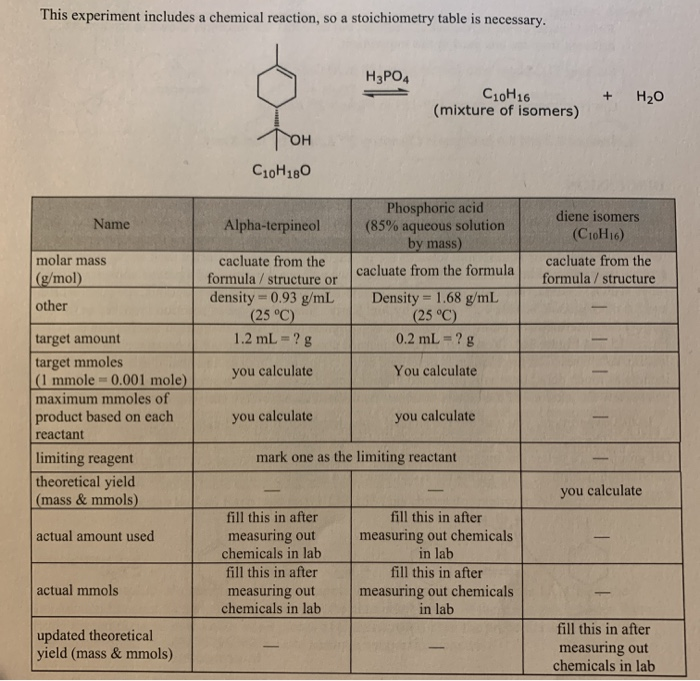please complete the stoichiometery table This experiment includes a chemical reaction, so a stoichiometry table is necessary. H3PO4 C10H16 (mixture of isomers) + H20 OH C20H180 Name Alpha-terpineol diene isomers (C16H16) molar mass (g/mol) Phosphoric acid (85% aqueous solution by mass) cacluate from the formula Density = 1.68 g/mL (25 °C) 0.2 mL = ? g cacluate from the formula / structure cacluate from the formula / structure or density = 0.93 g/mL (25 °C) 1.2 mL = ?g other...

• ### Isn't phosphoric acid corrosive, flammable, and explosive? I'm confused on how we are supposed to know...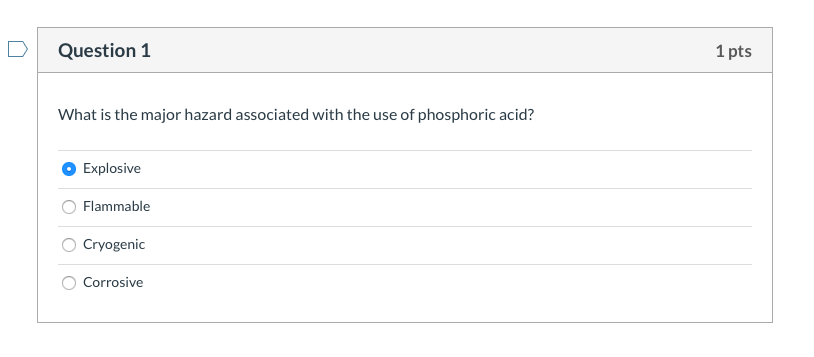Isn't phosphoric acid corrosive, flammable, and explosive? I'm confused on how we are supposed to know which one is "the major hazard". Were performing a gas chromatography experiment and so heat will be involved, maybe then the answer would be explosive? Question 1 1 pts What is the major hazard associated with the use of phosphoric acid? Explosive Flammable Cryogenic Corrosive

• ### 111 Experiment 11 AN INSTRUMENTAL ANALYSIS OF POP FOR ITS PHOSPHORIC ACID CONTENT The Pre-Lab ....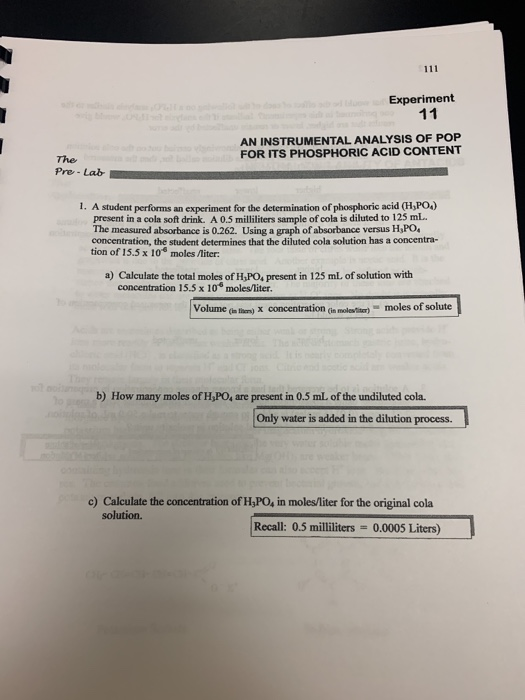111 Experiment 11 AN INSTRUMENTAL ANALYSIS OF POP FOR ITS PHOSPHORIC ACID CONTENT The Pre-Lab . A student performs an experiment for the determination of phosphoric acid (H)PO present in a cola soft drink. A 0.5 milliliters sample of cola is diluted to 125 mL. The measured absorbance is 0.262. Using a graph of absorbance versus H,PO concentration, the student determines that the diluted cola solution has a concentra- tion of 15.5 x 10 moles liter: a) Calculate the total...

• ### how do I find the theoretical yield of p-bromoacetanilide? Reagent Data! List of all reagents, catalysts...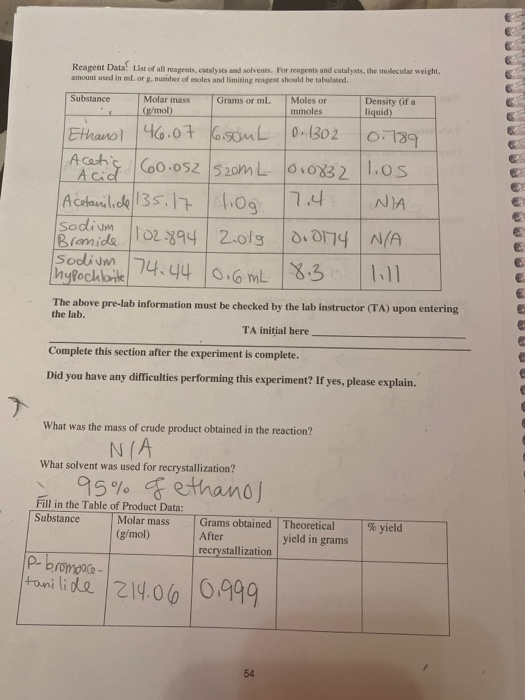how do I find the theoretical yield of p-bromoacetanilide? Reagent Data! List of all reagents, catalysts and solvents. For reagents and catalysts, the molecular weight, amount used in m or g. number of moles and limiting reagent should be tabelated. Substance Grams or ml. Molar mass (g/mol) Moles or mmoles Density (if a liquid) 0.1302 10.789 Ethanol 46.07 6.50mL | Acetic 60.052520mL I Acid 60. Acetanilide 135.17 1.00 sodium Bromide 1102.894 2.019 hypochlante 74.44 / 0.6 mL 01083) 17.4 1.os...

• ### QUESTION 10 To faciliate post-experiment data analysis, is is important to determine the limiting reagent in...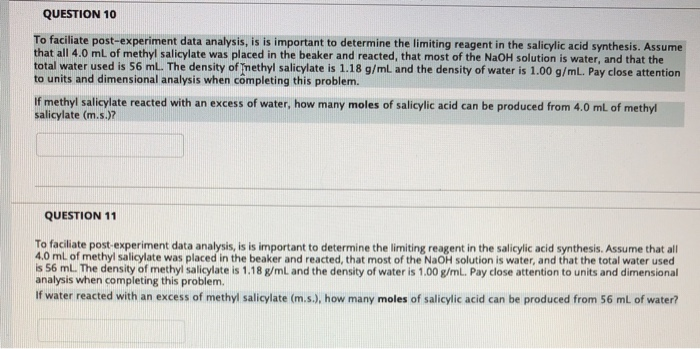QUESTION 10 To faciliate post-experiment data analysis, is is important to determine the limiting reagent in the salicylic acid synthesis. Assume that all 4.0 mL of methyl salicylate was placed in the beaker and reacted, that most of the NAOH solution is water, and that the total water used is 56 mL. The density of Tnethyl salicylate is 1.18 g/mL and the density of water is 1.00 g/mL. Pay close attention to units and dimensional analysis when cômpleting this problem....